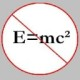You are currently browsing the daily archive for April 14, 2008.

[Update: Look for another (slicker) solution I found after coming up with the first one.]

My friend, John, asked me today if I had a solution to the following (well-known) problem which may be found, among other sources, in Chapter Zero (!) of the very famous book, Mathematical Circles (Russian Experience).

Three tablespoons of milk from a glass of milk are poured into a glass of tea, and the liquid is thoroughly mixed. Then three tablespoons of this mixture are poured back into the glass of milk. Which is greater now: the percentage of milk in the tea or the percentage of tea in the milk?

Note that there is nothing special about transferring three tablespoons of milk and/or tea from one glass to another – the problem doesn’t really change if we transfer one tablespoon of milk/tea instead, and that there is nothing special about transferring “volumes” – we could instead keep a count of, say, the number of molecules transferred.  We may, therefore, pose ourselves the following analogous “discrete” problem whose solution provides more “insight” into what’s really going on.

Jar W contains$n$ white objects (and no other objects) and jar B contains$n$ black objects (and no other objects.) We transfer$k$ objects from jar W to jar B. We then thoroughly mix –  in fact, we don’t have to – the contents of jar B, following which we transfer$k$ objects, this time, from jar B to jar W. Which is greater now: the percentage of black objects in jar W or the percentage of white objects in jar B?

Solution 1: Let us keep track of the number of black and white objects in both the jars before and after the transfers of$k$ objects from one jar to another. So, initially, in jar W,

# of white objects =$n$, and # of black objects =$0 \,$.

Also, in jar B,

# of white objects =$0 \,$, and # of black objects =$n$.

Now, we transfer$k$ objects from jar W to jar B. So, in jar W,

# of white objects =$n-k$, and # of black objects =$0 \,$.

Also, in jar B,

# of white objects =$k$, and # of black objects =$n$.

Finally, we transfer$k$ objects from jar B to jar W. Let the number of white objects out of those$k$ objects be$k_1$. Then, the number of black objects transferred equals$k-k_1$. Therefore, now, in jar W,

# of white objects =$n-k + k_1$, and # of black objects =$k-k_1$.

Also, in jar B,

# of white objects =$k-k_1$, and # of black objects =$n - (k-k_1)$.

From here, it is easy to see that the percentage of black objects in jar W is the same as the percentage of white objects in jar B! And, we are done.

Solution 2: (I think this is a slicker one, and I found it after pondering a little over the first solution I wrote above!) This one uses the idea of invariants, and there are, in fact, two of ’em in this problem! Note that at any given time,

# of white objects = # of black objects =$n$.

The above is the first invariant.

Also, note that after we transfer$k$ objects from jar W to jar B and then$k$ objects from jar B to jar W, the number of objects in each jar is also$n$. This is the second invariant. And, now the problem is almost solved!

Suppose, after we do the transfers of$k$ objects from jar W to jar B and then from jar B to jar W, the # of white objects in jar W is$k$. Then it is easy to see that the # of black objects in jar W is$n-k$ (using the second invariant mentioned above.) Similarly, using the first invariant, the # of white objects in jar B =$n-k$. Therefore, using the second invariant again, the # of black objects in jar B =$n - (n-k) = k$. And, from this the conclusion immediately follows!

### Blog Stats

• 357,717 hits

 Convictions ·… on Continued fraction for eJohn Favors on Solution to POW-13: Highly…Wayne J. Mann on Solution to POW-12: A graph co…erneststephen on The 54th Carnival of Math…anhtraisg on p^q + q^p is primeprof dr drd horia or… on My first postprof drd horia orasa… on My first postprof dr mircea orasa… on Inequality with lognotedscholar on Self-referential Paradoxes, In…prof dr mircea orasa… on Inequality with logprof dr mircea orasa… on Inequality with logprof dr mircea orasa… on 2010 in reviewkenji on Basic category theory, Iprof dr mircea orasa… on Solution to POW-10: Another ha…prof drd horia orasa… on Continued fraction for e